# BITSAT Mathematics Application Of Derivatives MCQs

Refer to BITSAT Mathematics Application Of Derivatives MCQs provided below. BITSAT Full Syllabus Mathematics MCQs with answers available in Pdf for free download. The MCQ Questions for Full Syllabus Mathematics with answers have been prepared as per the latest syllabus, BITSAT books and examination pattern suggested in Full Syllabus by BITSAT, NCERT and KVS. Multiple Choice Questions for Application Of Derivatives are an important part of exams for Full Syllabus Mathematics and if practiced properly can help you to get higher marks. Refer to more Chapter-wise MCQs for BITSAT Full Syllabus Mathematics and also download more latest study material for all subjects

## MCQ for Full Syllabus Mathematics Application Of Derivatives

Full Syllabus Mathematics students should refer to the following multiple-choice questions with answers for Application Of Derivatives in Full Syllabus. These MCQ questions with answers for Full Syllabus Mathematics will come in exams and help you to score good marks

### Application Of Derivatives MCQ Questions Full Syllabus Mathematics with Answers

#### Question: If f (x) = xx, then f (x) is increasing in interval :

• a) [0, e]
• b)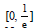• c) [0, 1]
• d) None of these#### Question: A cylindircal gas container is closed at the top and open at the bottom. if the iron plate of the top istime as thick as the plate forming the cylindrical sides. The ratio of the radius to the height of the cylinder using minimum material for the same capacity i

• a)• b)• c)• d)#### Question: The set of all values of a for which the function f(x) = (a2 – 3a + 2) (cos2x/4 –sin2x/4) + (a –1) x + sin 1does not possess critical points is

• a) [1, ∞)
• b)• c)  (–2, 4)
• d)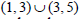#### Question: Match List I with List II and select the correct answer using the code given below the lists:• a) (A) (B) (C) (D)

1     4    5   3

• b) (A) (B) (C) (D)

1    3    5   4

• c)   (A) (B) (C) (D)

5   4    2    3

• d) (A) (B) (C) (D)

5    3    2   4

5   4    2    3

#### Question:  What is the x-coordinate of the point on the curve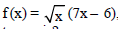where the tangent is parallel to x-axis?

• a)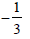• b)• c)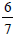• d)#### Question: A wire 34 cm long is to be bent in the form of a quadrilateral of which each angle is 90°. What is the maximum area which can be enclosed inside the quadrilateral?

• a) 68 cm2
• b) 70 cm2
• c) 71.25 cm2
• d) 72. 25 cm2

#### Question: Consider the following statements in respect of the function

f (x) = x3 – 1, xÎ[-1,1]

I. f (x) is increasing in [– 1, 1]

II. f (x) has no root in (– 1, 1).

Which of the statements given above is/are correct?

• a) Only I
• b) Only II
• c) Both I and II
• d) Neither I nor II

#### Question: At an extreme point of a function f (x), the tangent to the curve is

• a) Parallel to the x-axis
• b) Perpendicular to the x-axis
• c) Inclined at an angle 45° to the x-axis
• d) Inclined at an angle 60° to the x-axis

#### Question: The curve y = xex has minimum value equal to

• a)• b)• c)

– e

• d) e#### Question: The line which is parallel to X-axis and crosses the curve y = √x at an angle of 45°, is

• a)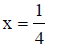• b)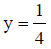• c)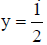• d) y = 1#### Question: Tangents are drawn from the origin to the curve y = cos x. Their points of contact lie on

• a) x2y2 = y2 – x2
• b) x2y2 = x2 + y2
• c) x2y2 = x2 – y2
• d) None of these

Answer: x2y2 = x2 – y2

• a) 0
• b) π
• c) 2π
• d) 3π/2

• a) x = 2
• b) x = –2
• c) x = 0
• d) x = 1

#### Question: If a and b are non-zero roots of x2 + ax + b = 0 then the least value of x2 + ax + b is

• a)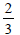• b)• c)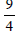• d) 1#### Question: If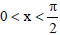, then

• a) tan x < x < sin x
• b) x < sin x < tan x
• c) sin x < tan x < x
• d) None of these

#### Question: The interval in which the function 2x3 + 15 increases less rapidly than the function 9x2 – 12x, is –

• a) (–∞, 1)
• b) (1, 2)
• c) (2, ∞)
• d) None of these

• a) 10
• b) 20
• c) 30
• d) 40

#### Question: The equation of all lines having slope 2 which are tangent to the curve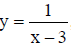x≠3 is

• a) y = 2
• b) y = 2x
• c) y = 2x + 3
• d) None of these

#### Question: The function f (x) = (x(x–2))2 is increasing in the set

• a)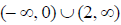• b) (– ∞, 1)
• c)• d) (1, 2)#### Question: If a2 x4 + b2 y4 = c4, then the maximum value of xy is

• a)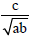• b)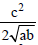• c)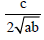• d)#### Question: If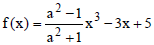is a decreasing function of x in R then the set of possible values of a (independent of x) is

• a) (1, ∞)
• b) (-∞, -1)
• c) [-1, 1]
• d) None of these

#### Question: The diagonal of a square is changing at the rate of 0.5 cm/sec. Then the rate of change of area, when the area is 400 cm2, is equal to

• a)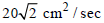• b) 10 √2 cm2 / sec
• c)• d)Answer: 10 √2 cm2 / sec

• a) –1
• b) – 3/4
• c) 4/3
• d) 1

#### Question: The function f(x) = sin x – kx – c, where k and c are constants, decreases always when

• a) k > 1
• b)• c) k < 1
• d)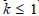#### Question: The minimum value of f (x) = sin4 x + cos4 x in the intervalis

• a)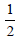• b) 2
• c) √2
• d) 1• a) (1, 1)
• b) (0, 1)
• c) (1, 0)
• d) No point

#### Question: The function f(x) = 2x3 – 3x2 – 12x + 4, has

• a) Two points of local maximum
• b) Two points of local minimum
• c) One maxima and one minima
• d) No maxima or minima

Answer: One maxima and one minima

• a) 4 π
• b) 2 π
• c) 6 π
• d) 3 π

#### Question: The function f(x) = tan x – 4x is strictly decreasing on

• a)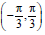• b)• c)• d)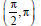• a) –1
• b) 1
• c) 0
• d) 2

• a) 0
• b) 2
• c) 8
• d) 9

#### Question: The value of a in order that f (x) = sin x – cos x – ax + b decreases for all real values is given by

• a)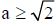• b) a < √2
• c)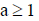• d) a < 1#### Question: The equation of tangent to the curve y = sin x at the point (π, 0) is

• a) x + y = 0
• b) x + y = π
• c) x – y =π
• d) x – y = 0

Answer: x + y = π

#### Question: If f(x) = cos x, then

• a) f(x) is strictly decreasing in (0, π)
• b) f(x) is strictly increasing in (0, 2π)
• c) f(x) is neither increasing nor decreasing in (π, 2π)
• d) All the above are correct

Answer:  f(x) is strictly decreasing in (0, π)

#### Question: The greatest value of f (x) = cos (xe[x] + 7x2 – 3x),x £ [–1, ∞) is –

• a) –1
• b) 1
• c) 0
• d) None of these

• a) (1,∞)
• b) (–1,∞)
• c) (–∞, ∞)
• d) (0, ∞)

• a) 116
• b) 126
• c) 136
• d) 416

#### Question: If at any point S of the curve by2 = (x + a)3, the relation between subnormal SN and subtangent ST be p (SN) = q (ST)2 then p/q is equal to

• a) 8b/27
• b) 8a/27
• c) b/a
• d) None of these

#### Question: The equation of one of the tangents to the curve y = cos(x +y),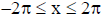that is parallel to the line x + 2y = 0, is

• a) x + 2y = 1
• b) x + 2y = π/2
• c) x + 2y = π/4
• d) None of these

Answer: x + 2y = π/2

#### Question: For all values of x, function f (x) = 2x3 + 6x2 + 7x – 19 is

• a) Monotonic increasing
• b) Monotonic decreasing
• c) Not monotonic
• d) None of these

#### Question: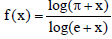is

• a) increasing in [0,∞)
• b) Decreasing in [0, ∞)
• c)• d)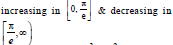• a) -2
• b) -1
• c) 2
• d) 4

• a) λ > 1/2
• b) λ < 1/2
• c) λ < 2
• d) λ > 2

• a) (1, 1)
• b) (0, 1)
• c) (1, 0)
• d) No point

• a) -1
• b) 1
• c) 0
• d) 2

## More Study Material

### BITSAT Full Syllabus Mathematics Application Of Derivatives MCQs

We hope students liked the above MCQs for Application Of Derivatives designed as per the latest syllabus for Full Syllabus Mathematics released by BITSAT. Students of Full Syllabus should download the Multiple Choice Questions and Answers in Pdf format and practice the questions and solutions given in above Full Syllabus Mathematics MCQs Questions on daily basis. All latest MCQs with answers have been developed for Mathematics by referring to the most important and regularly asked topics which the students should learn and practice to get better score in school tests and examinations. Studiestoday is the best portal for Full Syllabus students to get all latest study material free of cost.

### MCQs for Mathematics BITSAT Full Syllabus Application Of Derivatives

Expert teachers of studiestoday have referred to NCERT book for Full Syllabus Mathematics to develop the Mathematics Full Syllabus MCQs. If you download MCQs with answers for the above chapter daily, you will get higher and better marks in Full Syllabus test and exams in the current year as you will be able to have stronger understanding of all concepts. Daily Multiple Choice Questions practice of Mathematics and its study material will help students to have stronger understanding of all concepts and also make them expert on all critical topics. You can easily download and save all MCQs for Full Syllabus Mathematics also from www.studiestoday.com without paying anything in Pdf format. After solving the questions given in the MCQs which have been developed as per latest course books also refer to the NCERT solutions for Full Syllabus Mathematics designed by our teachers

#### Application Of Derivatives MCQs Mathematics BITSAT Full Syllabus

All MCQs given above for Full Syllabus Mathematics have been made as per the latest syllabus and books issued for the current academic year. The students of Full Syllabus can refer to the answers which have been also provided by our teachers for all MCQs of Mathematics so that you are able to solve the questions and then compare your answers with the solutions provided by us. We have also provided lot of MCQ questions for Full Syllabus Mathematics so that you can solve questions relating to all topics given in each chapter. All study material for Full Syllabus Mathematics students have been given on studiestoday.

#### Application Of Derivatives BITSAT Full Syllabus MCQs Mathematics

Regular MCQs practice helps to gain more practice in solving questions to obtain a more comprehensive understanding of Application Of Derivatives concepts. MCQs play an important role in developing understanding of Application Of Derivatives in BITSAT Full Syllabus. Students can download and save or print all the MCQs, printable assignments, practice sheets of the above chapter in Full Syllabus Mathematics in Pdf format from studiestoday. You can print or read them online on your computer or mobile or any other device. After solving these you should also refer to Full Syllabus Mathematics MCQ Test for the same chapter

#### BITSAT MCQs Mathematics Full Syllabus Application Of Derivatives

BITSAT Full Syllabus Mathematics best textbooks have been used for writing the problems given in the above MCQs. If you have tests coming up then you should revise all concepts relating to Application Of Derivatives and then take out print of the above MCQs and attempt all problems. We have also provided a lot of other MCQs for Full Syllabus Mathematics which you can use to further make yourself better in Mathematics

Where can I download latest BITSAT MCQs for Full Syllabus Mathematics Application Of Derivatives

You can download the BITSAT MCQs for Full Syllabus Mathematics Application Of Derivatives for latest session from StudiesToday.com

Can I download the MCQs of Application Of Derivatives Full Syllabus Mathematics in Pdf

Yes, you can click on the links above and download topic wise MCQs Questions PDFs for Application Of Derivatives Full Syllabus for Mathematics

Are the Full Syllabus Mathematics Application Of Derivatives MCQs available for the latest session

Yes, the MCQs issued by BITSAT for Full Syllabus Mathematics Application Of Derivatives have been made available here for latest academic session

How can I download the Application Of Derivatives Full Syllabus Mathematics MCQs

You can easily access the links above and download the Application Of Derivatives Full Syllabus MCQs Mathematics for each topic

Is there any charge for the MCQs with answers for Full Syllabus Mathematics Application Of Derivatives

There is no charge for the MCQs and their answers for Full Syllabus BITSAT Mathematics Application Of Derivatives you can download everything free

How can I improve my MCQs in Full Syllabus Mathematics Application Of Derivatives

Regular revision of MCQs given on studiestoday for Full Syllabus subject Mathematics Application Of Derivatives can help you to score better marks in exams

What are MCQs for Full Syllabus Mathematics Application Of Derivatives

Multiple Choice Questions (MCQs) for Application Of Derivatives Full Syllabus Mathematics are objective-based questions which provide multiple answer options, and students are required to choose the correct answer from the given choices.